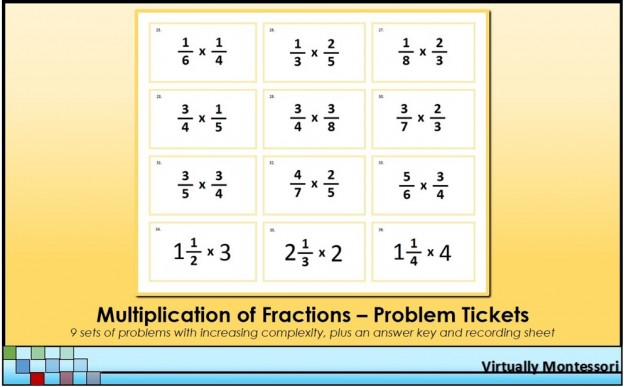# Multiplication of Fractions Problem Tickets

These fraction problems are intended to be follow-up practice after a lesson. Each group of problems adds a new layer of complexity (see bulleted list below for how each problem set is differentiated). The yellow border represents the multiplication operation in Montessori math lessons and materials.
The following items are included in this material:

• Multiplication of fractions problems tickets
• Problems #1-6: Multiplication of a fraction by a whole number
• Problems #7-9: Commutative property of multiplication (referring to problems #4-6)
• Problems #10-18: Multiplying a whole number by a fraction
• Problems #19-33: Multiplying a fraction by a fraction
• Problems #34-42: Multiplying mixed numbers: mixed number in multiplicand
• Problems #43-51: Multiplying mixed numbers: mixed number in multiplier
• Problems #52-59: Multiplying mixed numbers: mixed numbers in multiplicand & multiplier
• Problems #60-67: Cancelling and factoring out with fractions
• Problems #68-72: Multiplying three fractions